• Matlab关于对图像深度的处理，只需在源代码中换掉图片即可，相当方便，亲测有效哦，关于图像处理的。
• 数据可视化 要使用plot函数来绘制图形，需要执行以下步骤： 通过指定要绘制函数的变量x的值的范围来定义x。 定义函数，y = f(x) 调用plot命令，如下：plot(x，y) 以下示例将演示该概念。下面绘制x的值范围是从0到...
数据可视化
要使用plot函数来绘制图形，需要执行以下步骤：
通过指定要绘制函数的变量x的值的范围来定义x。定义函数，y = f(x)调用plot命令，如下：plot(x，y)
以下示例将演示该概念。下面绘制x的值范围是从0到100，使用简单函数y = x，增量值为5。 创建脚本文件并键入以下代码 - x = [0:5:100]; y = x; plot(x, y) 在图上添加标题，标签，网格线和缩放 MATLAB允许沿x轴和y轴，网格线添加标题，标签，并且还可以调整轴来绘制图形。
xlabel和ylabel命令沿x轴和y轴生成标签。  title命令用于在图表上设置标题。  grid on命令用于将网格线放在图形上。  axis equal命令允许生成具有相同比例因子的绘图和两个轴上的空格。  axis square命令生成一个方形图。
x = [0:0.01:10]; y = sin(x); plot(x, y), xlabel(‘x’), ylabel(‘Sin(x)’), title(‘Sin(x) Graph’), grid on, axis equal
在同一个图上绘制多个函数

可以在同一个图上绘制多个图形。以下示例演示了这一概念 - 示例 创建脚本文件并键入以下代码 - x = [0 : 0.01: 10]; y = sin(x); g = cos(x); plot(x, y, x, g, ‘.-’), legend(‘Sin(x)’, ‘Cos(x)’) legend函数的基本用法是： LEGEND(string1,string2,string3, …) 分别将字符串1、字符串2、字符串3……标注到图中，每个字符串对应的图标为画图时的图标。
**在图上设置颜色**

MATLAB提供了八个基本的颜色选项来绘制图形。 下表显示了颜色及其代码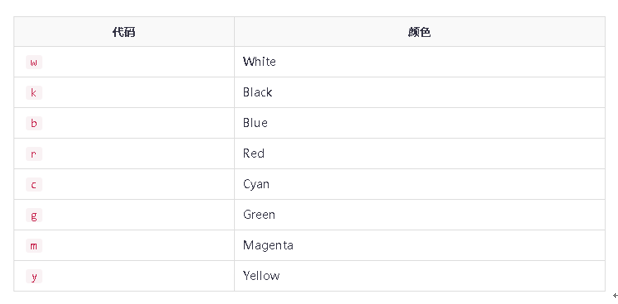设置轴刻度 axis命令用来设置轴刻度。可以通过以下方式使用axis命令为x和y轴提供最小和最大值： axis ( [xmin xmax ymin ymax] )
生成子图 当在同一个图中创建一个绘图数组时，这些图中的每一个图称为子图。 subplot命令用于创建子图。 该命令的语法是 ： subplot(m, n, p) 其中，m和n是绘图数组的行数和列数，p表示放置指定图形的位置。 使用subplot命令创建的每个曲线都可以有自己的特点。 以下示例演示了这一概念 - 示例 下面示例将生成两个区域块 -
创建脚本文件并键入以下代码 - x = [0:0.01:5]; y = exp(-1.5*x).sin(10x); subplot(1,2,1) plot(x,y), xlabel(‘x’),ylabel('exp(–1.5x)sin(10x)’),axis([0 5 -1 1]) y = exp(-2x).sin(10x); subplot(1,2,2) plot(x,y),xlabel(‘x’),ylabel(‘exp(–2x)*sin(10x)’),axis([0 5 -1 1]) MATLAB 执行上面示例代码，得到以下结果 -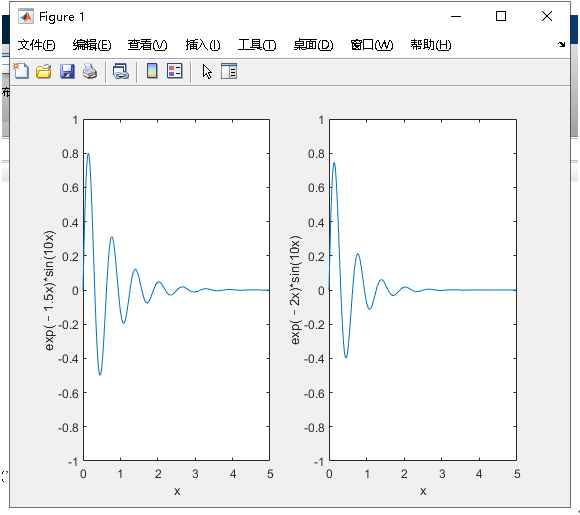绘制条形图 bar命令绘制二维条形图 示例 假设有10名学生，这些学生某次考试获得分数是：75,58,90,87,50,85,92,75,60和95，使用这此分数来绘制条形图如下。 创建脚本文件并键入以下代码 - x = [1:10]; y = [75, 58, 90, 87, 50, 85, 92, 75, 60, 95]; bar(x,y), xlabel(‘Student’),ylabel(‘Score’), title(‘First Sem:’) print -deps graph.eps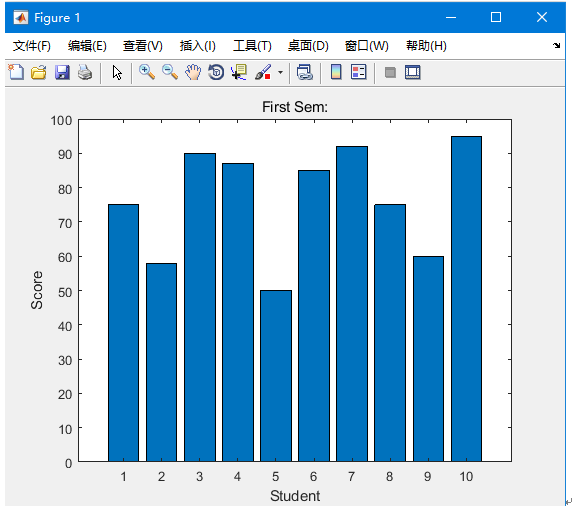绘制等高线 两个变量的函数的轮廓线是一个曲线，函数有一个恒定值。等高线用于通过连接等于高于某一水平的点(如平均海平面)来创建轮廓图。 MATLAB提供了绘制轮廓图的contour函数。 示例： contour(theta0_vals, theta1_vals, J_vals, logspace(-2, 3, 20)); %contour(X,Y,Z,v) 使用 X 和 Y 绘制 Z 的等高线图。V规定等高线的范围 %logspace(a,b,N) 把10的a次方到10的b次方区间分成N份
三维图 三维图基本上显示的是由两个变量g = f(x，y)中的函数定义的表面。 像之前一样，要定义g，首先使用meshgrid命令在函数的域上创建一组(x，y)点。 接下来，分配函数本身。 最后，使用surf命令创建一个曲面图。 surf(x, y, g)
展开全文plot函数
• 基于MATLAB中MatConvNet工具包实现的VGG网络特征和卷积核可视化
• matlab的egde源代码深度可视化 一些使用Caffe和Matlab的有趣的可视化演示。 FaceVis 可视化由人脸识别模型存储的人脸。 FilterVis 可视化CNN模型学习的中级功能。 启蒙主义 可视化CNN模型中的分类器神经元。 神经...
• 最近在尝试ViT模型，在可视化输入数据时需要把图片分割成若干部分（patches）。示例图片如下， 代码如下: %% load image uiopen(' earth.jpeg',1) earth_gray = rgb2gray(earth); % 转换成灰度图片 figure;imagesc...
前言
此文章会涉及常用的画图函数和技巧，包括1维/2维/3维数据。 文章会一直更新。
2维图片
图像分割成不同的patches
27.08.2021
最近在尝试ViT模型，在可视化输入数据时需要把图片分割成若干部分（patches）。示例图片如下，代码如下:
%% load image
uiopen(' earth.jpeg',1)
earth_gray = rgb2gray(earth);	% 转换成灰度图片
figure;imagesc(earth_gray)
whos earth_gray
%% cutout 	剪切
earth_gray_cutout = earth_gray;
earth_gray_cutout(1:30,:) = [];
earth_gray_cutout(end-10:end,:) = [];
earth_gray_cutout(:,1:50) = [];
earth_gray_cutout(:,end-20: end) = [];
figure;imagesc(earth_gray_cutout)
whos earth_gray_cutout

%% resize 大小变成 400 X 400
image_size = 400;
earth_gray_cutout_resize = imresize(earth_gray_cutout,[image_size image_size]);

%% divide the image into several patches
template_image = earth_gray_cutout_resize;

patch_size_given = 40;	% 每一部分的尺寸， 40个像素
number_of_patchs = image_size / patch_size_given;

figure;
for index_patch_1 = 1:number_of_patchs
for index_patch_2 = 1:number_of_patchs
patch_pickup = template_image(1 + (index_patch_1-1) * patch_size_given : index_patch_1*patch_size_given,...
1 + (index_patch_2-1) * patch_size_given : index_patch_2*patch_size_given);

imagesc(template_patchs); colormap hot; axis image
pause(0.02)
end
end


结果如下可以看到，图片已经被”分割“好了，但是坐标轴和刻度却影响了整体的效果。下面的代码可以去除不需要的信息。
% 移除坐标轴刻度和边框
set(gca,'Visible','off');	% 包括title

% 还有一种做法，可以保留title
set(gca,'XColor', 'none','YColor','none')


效果如下：1维数据
柱状图 histogram
在分析数据分布式，常常会用到树状图histogram。当需要对比不同的数据时，如果能同时画出两个或者更多个树状图，那么就可以进行非常直观的对比。 这里需要用到一个第三方函数brewermap
map = brewermap(2, 'Set1');	% 一系列颜色
data1 =rand(100000,1);
data2 =rand(100000,1)*0.5;
data3 = rand(100000,1)*1.5;
figure;
histogram(data1,'facecolor',map(1,:),'facealpha',.5,'edgecolor','none')
hold on
histogram(data2,'facecolor',map(2,:),'facealpha',.5,'edgecolor','none')
hold on
histogram(data3,'facecolor',map(3,:),'facealpha',.5,'edgecolor','none')

box off
axis tight
% legalpha('H1','H2','H3','location','northeast')
legend('A','B','C')
legend boxoff

结果如下: 三种数据分布都表达张一个图上。展开全文• 基于 Shotton 等人，单深度图像部分的实时人体姿态识别，CVPR，2011 文件： 1. makeclick.m-通过单击创建数据集2.makespiral.m --使用螺旋函数制作数据集3. rt1.m --用于种植森林的主要脚本4. branch.m -- 用于生长...
• 之前的博客中介绍过利用MATLAB可视化图像特征，因为最近在看《深度学习21天实战caffe》，里面有一章节是关于可视化的，所以把可视化mnist数据集的代码共享一下，在这里要感谢赵永科老师clc; clear; close all; image...
之前的博客中介绍过利用MATLAB可视化图像特征，因为最近在看《深度学习21天实战caffe》，里面有一章节是关于可视化的，所以把可视化mnist数据集的代码共享一下，在这里要感谢赵永科老师
clc;
clear;
close all;
image_file_name='t10k-images.idx3-ubyte';
index_file_name='t10k-labels.idx1-ubyte';

fid1=fopen(image_file_name,'rb');
fid2=fopen(index_file_name,'rb');

fclose(fid1);
fclose(fid2);

image_data=image_data(17:end);
index_data=index_data(9:end);
image_buffer=zeros(28,28);

for k=1:100:length(image_data)/28/28
figure(10000);
for t=1:10000
image_buffer=reshape(image_data((k+t-2)*28*28+1:(k+t-1)*28*28),28,28)';
subplot(100,100,t);
imshow(uint8(image_buffer)');
title(num2str(index_data(k+t-1)));
end
pause;
end
效果图如下：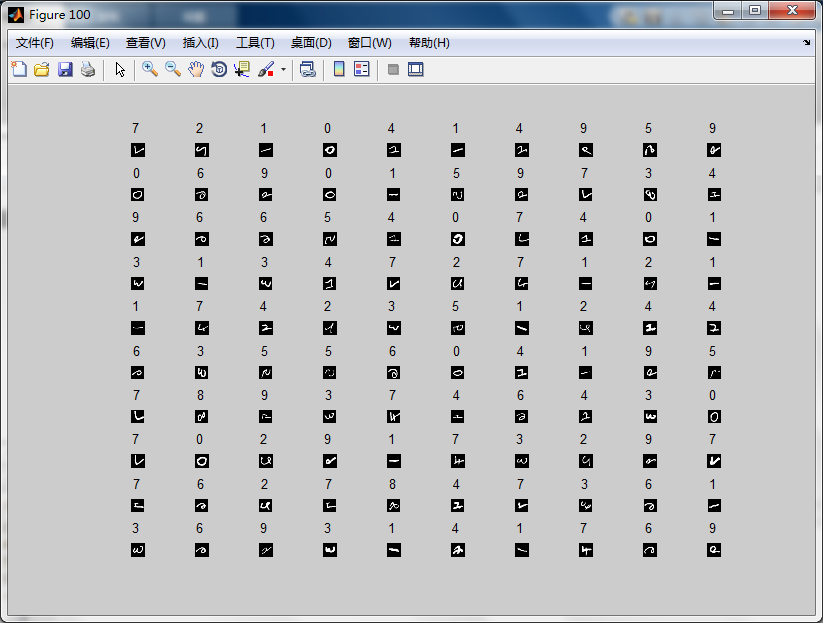在这里要感谢赵永科老师，代码是赵老师书里的。
参考文献：《深度学习21天实战caffe》赵永科
展开全文数据
• 前面博客中介绍了怎么样利用MATLAB实现特征可视化，那一篇博客中介绍了一个对权重可视化的博客，本来不打算写这个博客了，但是我仔细看了那一篇博客，有点繁琐，所以我又整理了一下用MATLAB实现模型参数权重可视...
前面博客中介绍了怎么样利用MATLAB实现特征图的可视化，那一篇博客中介绍了一个对权重可视化的博客，本来不打算写这个博客了，但是我仔细看了那一篇博客，有点繁琐，所以我又整理了一下用MATLAB实现模型参数权重可视化的部分，这一篇介绍对第一个卷积层权重可视化，下一篇博客介绍Alexnet中2到5卷积层的可视化，请大家注意，只有卷积层才能可视化。  通过对训练后的模型进行可视化可以判断模型的优劣，怎么判断直接看博文后面的效果图，一看便知。直接放MATLAB代码，基于Alexnet，模型是前面介绍内容训练出来的模型，这个模型训练的不好，从参数权重图上就可以看出来，最后我会做一个好的和坏的第一个卷积层的参数可视化效果图，用于对比，如果想要可视化其它网络模型，只需要修改相应的部分就行，这里不再赘述。
clc;
clear;
close all;
caffe.set_mode_cpu();
net=caffe.Net('alexnetdeploy.prototxt','cloth_iter_100000.caffemodel','test');
net.layer_names      %显示都有哪些层
net.blob_names
conv1_layer=net.layer_vec(1);
blob1=conv1_layer.params(1);    %注意只有卷积层是有参数的
w=blob1.get_data();
size(w)
W=zeros(11*3,11*96);
for u=1:3
for v=1:96
W(11*(u-1)+(1:11),11*(v-1)+(1:11))= w(:,:,u,v)';
end
end
W=W-min(min(W));
W=W/(max(max(W)))*255;     %从0-1转换到255
W=uint8(W);
W=[W,zeros(size(W,1),4*11)];
WW=cat(3,W(1:11,:),W(12:22,:),W(23:33,:));
W=zeros(10*12,10*12,3);
for u=1:10
for v=1:10
W((u-1)*12+(1:11),(v-1)*12+(1:11),:)=WW(:,(u-1)*11*10+(v-1)*11+(1:11),:);
end
end
W=uint8(W);
figure;
imshow(W);

效果图：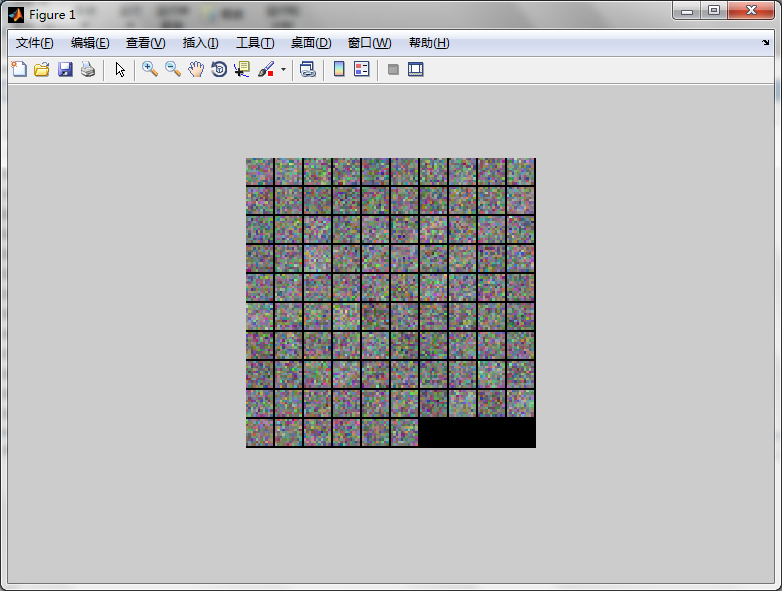上面这是一个不好的效果图，因为图案类似噪音太严重，不够平滑，不够美观。这一张权重图是bvlc_reference_caffenet.caffemodel的权重图，这个效果图比较平滑，也可以观察到一部分权重负责提取高频灰度特征，而另一些负责提取低频彩色特征。哪个好哪个坏，一看便知，这也是可视化权重的一个重要作用。
展开全文• %判断是否是RGB图像老版本的matlab可以使用 J001=rgb2gray(I001);%转换为灰度 subplot(121);imshow(I001);title('彩色图像'); subplot(122);imshow(J001);title('灰度图像'); K001R(:,:,:,1)=I001; K001R(:,:,:,2)...计算机视觉
• CIFAR10数据集共有matlab、python、及C语言三种类型的数据集，我下载的是matlab版本，下载完之后显示的是矩阵集合形式而非图片的形式，在网络上搜寻方法并理解代码如下：load(['yourpath']); for i = 1:size(data,1)...cifar10 数据
• ## matlab将深度图转为点云

万次阅读 热门讨论 2018-10-09 10:58:25
matlab深度图转为点云显示的代码： intrinsic=[fx,0,cx  0,fy,cy  0,0,1]; fdx=intrinsic(1,1); fdy=intrinsic(2,2); u0=intrinsic(1,3); v0=intrinsic(2,3); [h,w] = size(imdepth); u=repmat...
• matlab图像均衡化的代码从RGB图像进行高光谱重建以实现静脉可视化 我们提出了一种数据驱动的方法来从RGB图像中重建高光谱图像。 该方法基于残差学习方法，该方法可有效捕获数据流形的结构，并考虑到存在于RGB图像中...
• 利用Matlab将mnist数据可视化准备 安装MATLAB2014A LINUX 版 get_mnist.sh下载mnist数据集文件 步骤 Matlab 代码（show_mnist_data.m,可以放在\$CAFFE_ROOT/data/mnist/下，或者其他包含mnist数据文件的目录下运行 ...caffe mnist
• 在《多媒体技术原理》教学中,如何讲解傅里叶变换...本文借助于可视化MATLAB,将抽象的理论问题图形化、具体化,不仅能大幅度地降低学生理解的难度、提升学生理解的速度,还能加深学生理解的深度,从而达到更好的教学效果。
• CNN可视化工具：用于深度卷积神经网络中的单元可视化的工具包 介绍 该存储库包含用于可视化深CNN内的单元（或称为神经元或卷积过滤器）的代码和结果。 一些代码已用于深度场景CNN中出现的物体检测器》。 您可以将此...
• 上一篇博客介绍了如果可视化第一个卷积层的卷积核，可视化的一个重要作用就是判断训练权重的好坏，效果可以参考我上一篇博客，这篇博客介绍把卷积核可视化写成MATLAB的一个函数，用来直接调用就可以实现卷积核权重...
• 您可以任意指定感兴趣的变量、深度、要从结果中排除的变量（因为绘制所有变量会使输出不可读），用不同的颜色标记指定的变量等。 该声明 A(B(1:N)) = func(a,b,c) + exp(D) 在依赖中创建边和节点 A <- B, ...
• 该代码旨在可视化水下温度数据的垂直结构。 此代码专门设计用于使用由普渡大学和伊利诺伊州-印第安纳州 SeaGrant（NDBC 站 45170）拥有和运营的密歇根湖浮标收集的数据。 您可以选择将动画保存为 .gif 图像。 温度 ...
• [关键词]图像处理、IPCV 演示、深度学习、深度学习、迁移学习、介绍、对象识别、图像分类、计算机视觉、神经网络、人工智能、视觉检测、可视化 单击此处获取类激活映射为判别性定位学习深度特征麻省理工学院的...
• 使用网络分析仪• 可视化和理解网络架构， • 检查您是否正确定义了架构， •在训练之前发现问题。 分析网络检测到的问题包括• 缺失或断开的层， • 层输入的大小不匹配或不正确， • 层输入数不正确， • 无效的...
• 针对于图像来说，训练好一个模型后，需要比较特征差异或者做分析的时候，需要将模型进行可视化，这样能够无更加直观的分析深度学习中特征的变化。能够与其他网络提取的特征进行观察，分析差异。 代码： import cv2 ...python
• CNN卷积神经网络Matlab实现例程，使用了matlabR2019a自带的深度学习工具箱,一维数据分类，二维cnn
• 这是将KITTI数据集的稀疏深度图绘制为单眼深度估计任务训练或结果可视化的简单实现。 要求 这些代码已经在Ubuntu 16.04 LTS上使用Python 2.7和Matlab-linux进行了测试。 数据准备 对于用于训练任务或结果可视化的...
• 深度图，以及 光流数据。 用法示例（在Python脚本中）： from iminspect . inspector import inspect , DataType import numpy as np # Show random noise image: inspect ( np . random . rand ( 4096 , 4096 ) - ...
• 摘要：本文详细介绍MATLAB R2019b的安装步骤，为方便安装这里提供了完整安装文件的百度网盘下载链接供大家使用。从文件下载到证书安装本文都给出了每个步骤的截图，按照图示进行即可轻松完成安装使用。本文目录包括...安装教程
• 小编最近写文章的时候发现，训练网络，使用tensorboard进行可视化展示出来的精度曲线下载后生成csv或json类型的文件，小编尝试了使用各种方法进行文件的读取和绘图，但出来的效果并不能很好的展示在文章上面。...
• % % 此功能提供了一种用于可视化 3D 的改进技术% 向量场。 它依赖于单个补丁的有效使用% 呼叫和灯光效果以提供深度提示。 % % QUIVER3D(X,Y,Z,U,V,W) 将速度矢量绘制为带有分量的箭头% (u,v,w) 在点 (x,y,z)。 矩阵...
• 解析日志文件并绘制loss和accuracy曲线 将上一步得到的日志文件train_log.txt的完整路径输入下面Matlab脚本定义的PlotLossAndAccuracy()函数，即可解析并绘制loss和accuracy曲线 function result=...caffe
• 上一篇博客中介绍了如何使用MATLAB训练和测试数据，这篇博客介绍如何从训练好的模型中提取图像特征，并介绍把卷积层特征可视化方法。 之前提取特征都是用python，尽管用python提取特征很方便，但是感觉MATLAB提取...windows caffe...

# matlab可视化深度图matlab 订阅